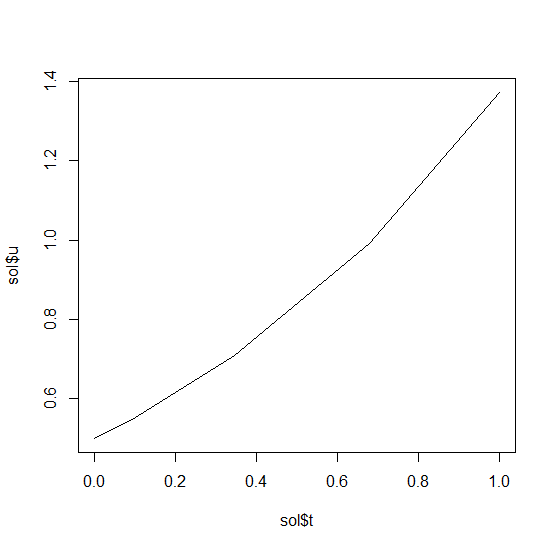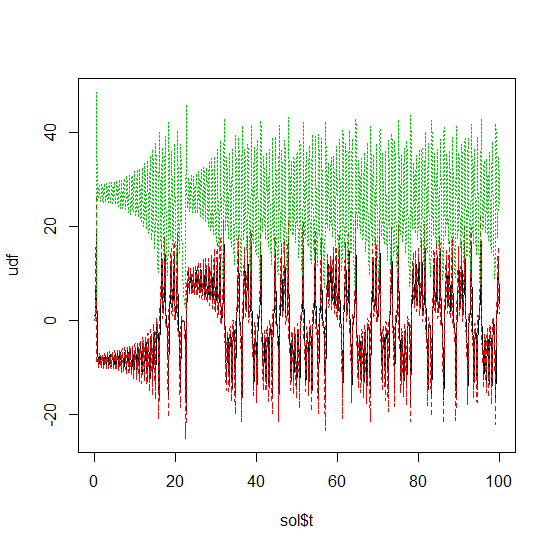# Solving Ordinary Differential Equations (ODE) in R with diffeqr

### 1D Linear ODEs

Let’s solve the linear ODE `u'=1.01u`. First setup the package:

``de <- diffeqr::diffeq_setup()``

Define the derivative function `f(u,p,t)`.

``````f <- function(u,p,t) {
return(1.01*u)
}``````

Then we give it an initial condition and a time span to solve over:

``````u0 <- 1/2
tspan <- c(0., 1.)``````

With those pieces we define the `ODEProblem` and `solve` the ODE:

``````prob = de\$ODEProblem(f, u0, tspan)
sol = de\$solve(prob)``````

This gives back a solution object for which `sol\$t` are the time points and `sol\$u` are the values. We can treat the solution as a continuous object in time via

and a high order interpolation will compute the value at `t=0.2`. We can check the solution by plotting it:

``plot(sol\$t,sol\$u,"l")``linear_ode

### Systems of ODEs

Now let’s solve the Lorenz equations. In this case, our initial condition is a vector and our derivative functions takes in the vector to return a vector (note: arbitrary dimensional arrays are allowed). We would define this as:

``````f <- function(u,p,t) {
du1 = p*(u-u)
du2 = u*(p-u) - u
du3 = u*u - p*u
return(c(du1,du2,du3))
}``````

Here we utilized the parameter array `p`. Thus we use `diffeqr::ode.solve` like before, but also pass in parameters this time:

``````u0 <- c(1.0,0.0,0.0)
tspan <- list(0.0,100.0)
p <- c(10.0,28.0,8/3)
prob <- de\$ODEProblem(f, u0, tspan, p)
sol <- de\$solve(prob)``````

The returned solution is like before except now `sol\$u` is an array of arrays, where `sol\$u[i]` is the full system at time `sol\$t[i]`. It can be convenient to turn this into an R matrix through `sapply`:

``mat <- sapply(sol\$u,identity)``

This has each row as a time series. `t(mat)` makes each column a time series. It is sometimes convenient to turn the output into a `data.frame` which is done via:

``udf <- as.data.frame(t(mat))``

Now we can use `matplot` to plot the timeseries together:

``matplot(sol\$t,udf,"l",col=1:3)``timeseries

Now we can use the Plotly package to draw a phase plot:

``plotly::plot_ly(udf, x = ~V1, y = ~V2, z = ~V3, type = 'scatter3d', mode = 'lines')``